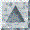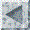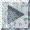“30” “30”](mstkla.md#MODEL%20ENTITY:)“30” “30”](Geom.md)“30” “30”](GeomRegion.md)“169” “131”

MODEL ENTITY OPERATORS:

GType GEnt_Type(PGeomEntity gent);

Type of a geometric model entity (GREGION, GFACE, GEDGE, GVERTEX,

GUNKNOWN).  Typically can be used in conjunction with the operator to

get mesh entity classification. So one can get the model entity a mesh

vertex is on, query what type it is and do something based on the

response (e.g. reposition the vertex only if it is classified in a

model region).

int GEnt_Number(PGeomEntity gent);

ID Number of geometric model entity. Can be called instead of

GR_Number, GF_Number, GE_Number and GV_Number.

int GEnt_OnBoundary(PGeomEntity g1, PGeomEntity g2);

Check if g2 is on the boundary of g1 (Check if a face is on the

boundary of a region, an edge is on the boundary of a face, a vertex

is on the boundary of a region)

int GEnts_Common(int nent, GType types, *PGeomEntity

gents, *int ncm, *GType ctypes, *PGeomEntity *cgents)

Given nent entitities (the entity pointers given in gents and their types in types), find their  common entities, cgents. The entities will be of the lowest order possible (but higher than the highest order of the input entities). For example, if we were given two model edges connected by one common face, the output would be this common face. On the other hand if these edges were not connected by a common face but the faces connected to them are on the boundary of the same model region, the model region would be returned as a common entity. Naturally, there may be more than one such common model entity in general geometric models.

int GEnt_OnExtBoundary(PGeomEntity gent)

Check if model entity is on external boundary“30” “30”](mstkla.md#MODEL%20ENTITY:)“30” “30”](Geom.md)“30” “30”](GeomRegion.md)